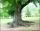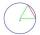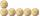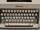# Circular arc

Calculate the length of the circular arc if the diameter d = 20cm and the angle alpha = 142 °

Result

x =  24.784 cm

#### Solution:Leave us a comment of example and its solution (i.e. if it is still somewhat unclear...):Be the first to comment!#### To solve this example are needed these knowledge from mathematics:

Need help calculate sum, simplify or multiply fractions? Try our fraction calculator.

## Next similar examples:

1. FlowerbedIn the park there is a large circular flowerbed with a diameter of 12 m. Jakub circulated him ten times and the smaller Vojtoseven times. How many meters each went by and how many meters did Jakub run more than Vojta?
2. Circle r,DCalculate the diameter and radius of the circle if it has length 52.45 cm.
3. Tree trunkCalculate the average tree trunk with a circumference of 149 cm.
4. Circle - simpleCalculate the area of a circle in dm2, if its circumference is 31.4 cm.
5. ChordPoint on the circle is the end point of diameter and end point of chord length of radius. What angle between chord and diameter?
6. Clock handsThe second hand has a length of 1.5 cm. How long does the endpoint of this hand travel in one day?
7. MineWheel in traction tower has a diameter 5 m. How many meters will perform an elevator cabin if wheel rotates in the same direction 49 times?
8. WellRope with a bucket is fixed on the shaft with the wheel. The shaft has a diameter 50 cm. How many meters will drop bucket when the wheels turn 15 times?
9. CoinHow many 50-cent coins must be put in a row that next 50-cent coins after them (above) rolled on it axis?
10. Obtuse angleWhich obtuse angle is creating clocks at 17:00?Convert magnitude of the angle α = 9°39'15" to radians:What is the radius of the circle whose perimeter is 6 cm?After driving 157 m bicycle wheel rotates 100 times. What is the radius of the wheel in cm?Two circles with a radius 4 cm and 3 cm have a center distance 0.5cm. How many common points have these circles?Before taking a typing course, Terrence could type 39 words per minute. By the end of the course, he was able to type 68 words per minute. Find the percent increase.Solve inequality: 3x + 6 > 14Three-quarters of an unknown number are 4/5. What is 5/6 of this unknown number?